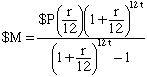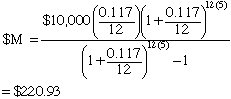Subject: interest rate

Hi, my name is anthony.

This question is base on my interest. I would like to know the formula for calculating this example: If you borrow \$10,000 from a bank with an APR of 11.7% to be paid off in 5 years, what is your monthly payment? Could you please show me step by step how this formula is developed. Thanks!

Hi Anthony,

I think that what you are looking for is called amortizing the loan. I want to illustrate the method with a smaller example. Suppose that I borrow \$1,000 for 6 months at an annual interest rate of 12%. I have calculated the monthly payments to be \$172.55. (I know that the calculation of the monthly payment is your question and I will return to it later.) The payment schedule is in the table below.

MonthPaymentInterestApplied to PrincipleUnpaid balance
1\$172.55\$10.00\$162.55\$837.45
2\$172.55\$8.37\$164.18\$673.27
3\$172.55\$6.73\$165.82\$507.45
4\$172.55\$5.07\$167.48\$339.97
5\$172.55\$3.40\$169.15\$170.82
6\$172.55\$1.70\$170.85\$0

The reasoning behind the schedule above is the following. At the first of month 1 I have borrowed \$1,000 at 12% per year. Hence in one month the interest owing will be one-twelth of 12% of the principle which is \$1,000. Thus the interest owing is  0.12/12 x \$1,000 = \$10.00. Hence \$10.00 of the \$172.55 payment goes toward the interest and the difference, \$162.55, is applied to the princple. Thus at the beginning of month 2 I owe \$1,000 - \$162.55 = \$837.45.

Thus in the second month I have borrowed \$837.45 and hence owe  0.12/12 x \$837.45 = \$8.37 in interest. Therefore \$8.37 of my \$172.55 payment goes to interest and the remainder, \$172.55 - \$8.37 = \$164.18 goes toward he principle. Thus at the beginning of the third month I owe \$837.45 - \$164.18 = \$673.27.

This procedure continues for 6 months when the unpaid balance is \$0. (The difference between \$170.82 and \$170.85 is roundoff error.)

The procudure to calculate the monthly payments for your loan of \$10,000 at 11.7% per year for 5 years is as follows. Assume that the payment is \$M per month. Complete the 60 rows in the table above that correspond to 5 years. In each of the 60 rows the Unpaid balance column will be an algebraic expression involving \$M. The expression in the Unpaid balance column and the sixtieth row must be zero so set the exprression equal to zero and solve for \$M. The result is the following formula for \$M.

If \$P is borrowed at an annual interest rate of r% for t years then the monthly payment \$M that satisfies the procedure above isFor your situation the monthly payment isCheers,
Penny
Go to Math Central